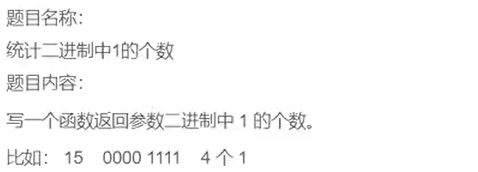::: tip 内存中存放的是补码，即计算一个数的补码的二进制序列中1的数量。

``````int count_bit_one(unsigned int a)
{
int count = 0;
while (a)
{
if (a % 2 == 1)
{
count++;
}
a = a / 2;
}
return count;
}

int main()
{
int a = 0;
scanf("%d", &a);
int count = count_bit_one(a);
printf("%d", count);
return 0;
}``````
``````-1
32``````

``````int count_bit_one(int a)
{
int count = 0;
int m = 0;
while (m < 32)
{
a >> m;
if (a & 1 == 1)
{
count++;
}
m++;
}
return count;
}

int main()
{
int a = 0;
scanf("%d", &a);
int count = count_bit_one(a);
printf("%d", count);
return 0;
}``````
``````-1
32``````

``````int count_bit_one(int a)
{
int count = 0;
while (a)
{
a = a & (a - 1);
count++;
}
return count;
}

int main()
{
int a = 0;
scanf("%d", &a);
int count = count_bit_one(a);
printf("%d", count);
return 0;
}``````

::: tip a = a & (a - 1)的写法，是将a的二进制数中每次去掉一个1。 如a=13（1011），a&(a-1)=1101&1100=1100，1100赋给a，相比于原来a的值1101，去掉了最后面的1。1100&1011=1000，1000赋给a，相比于原来a的值1100，再去掉最后的一个1。直到a的值变为0000，离开while循环，返回计数器。 :::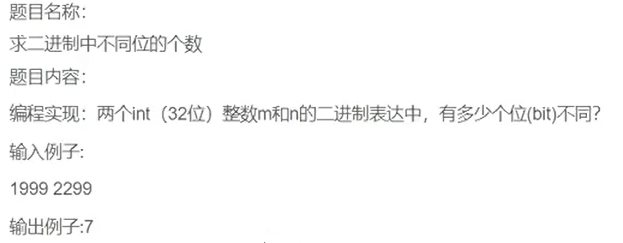上述题目思路可以将二进制数的每一位拿出来单独比较，但是代码效率不高。 可以从异或入手：

``````int count_diff_bit(m, n)
{
int tmp = m ^ n;
int count = 0;
while (tmp)
{
tmp = tmp & (tmp - 1);
count++;
}
}

int main()
{
int m, n;
scanf("%d %d",&m,&n);
int count = count_diff_bit(m, n);
printf("%d", count);
return 0;
}``````
``````1999 2299
7``````

``````void Print(int a)
{
int i = 0;  //向右移动的位置，从右往左0、2、4...
printf("奇数位是：");  //从右往左的1、3、5...
for(i = 30;i >= 0;i -= 2)  //最后一位奇数无需移动（移动0位），即i最小为0
//打印时候从左往右，从右往左计数第31位是最后一个奇数位，需要向右移动30位才能到达最后一位1的位置
{
printf("%d ", (a>>i)&1);
}
printf("\n");
printf("偶数位是：");  //从右往左的2、4、6...
for (i = 31; i >= 1; i -= 2)  //最后一位偶数需要向右移动1位，即i最小为1
//打印时候从左往右，从右往左计数第32位是最后一个偶数位，需要向右移动31位才能到达最后一位1的位置
{
printf("%d ", (a >> i) & 1);
}
}

int main()
{
int a = 0;
scanf("%d",&a);
int m = 0;
Print(a);
return 0;
}``````
``````150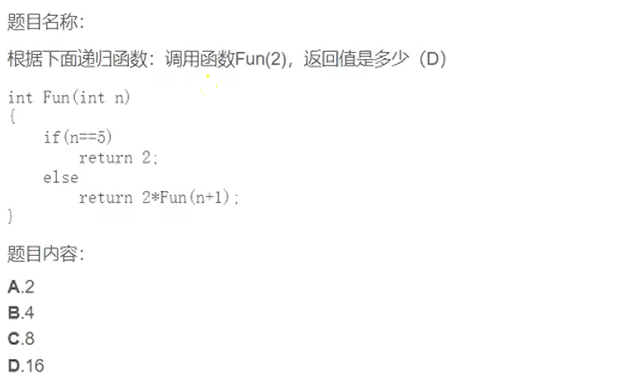上述代码反复调用得到Fun函数中n=5，返回2，即Fun函数的结果是2，一级一级×2返回，直到最后一层返回16。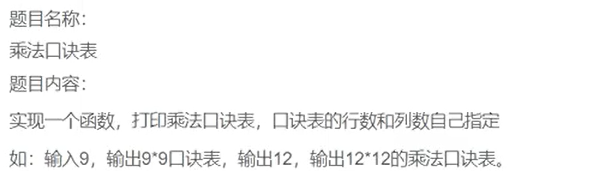``````void print(n)
{
int i = 0;
for (i = 0; i <= n; i++)
{
int j = 0;
for (j = 0; j <= i; j++)
{
printf("%d*%d=%-3d ",i,j,i*j);
}
printf("\n");
}
}

int main()
{
int n = 0;
scanf("%d",&n);
print(n);
return 0;
}``````
``````9
0*0=0
1*0=0   1*1=1
2*0=0   2*1=2   2*2=4
3*0=0   3*1=3   3*2=6   3*3=9
4*0=0   4*1=4   4*2=8   4*3=12  4*4=16
5*0=0   5*1=5   5*2=10  5*3=15  5*4=20  5*5=25
6*0=0   6*1=6   6*2=12  6*3=18  6*4=24  6*5=30  6*6=36
7*0=0   7*1=7   7*2=14  7*3=21  7*4=28  7*5=35  7*6=42  7*7=49
8*0=0   8*1=8   8*2=16  8*3=24  8*4=32  8*5=40  8*6=48  8*7=56  8*8=64
9*0=0   9*1=9   9*2=18  9*3=27  9*4=36  9*5=45  9*6=54  9*7=63  9*8=72  9*9=81``````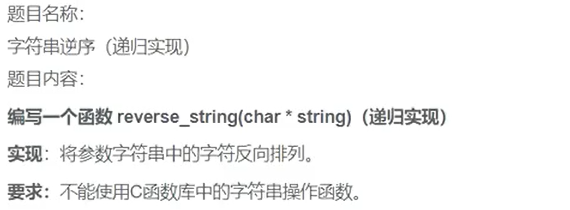使用C语言库函数的解法（不符合题目要求）：

``````#include <string.h>
void reverse(char arr[])
{
int left = 0;
int right = strlen(arr) - 1;
int tmp = 0;
while (left < right)
{
tmp = arr[left];
arr[left] = arr[right];
arr[right] = tmp;
left++;
right--;
}
}

int main()
{
char arr[] = "abcdef";
reverse(arr);
printf("%s\n",arr);
return 0;
}``````
``fedcba``

``````int my_strlen(char* str)
{
int count = 0;
while (*str != '\0')
{
count++;
str ++;
}
return count;
}

void reverse(char arr[])
{
char tmp = arr;
int len = my_strlen(arr);
arr = arr[len - 1];
arr[len - 1] = '\0';
if (my_strlen(arr + 1) > 1)  //arr是指针，函数中char arr[]可以写成char* arr
{
reverse(arr + 1);
}
arr[len - 1] = tmp;
}

int main()
{
char arr[] = "abcdef";
reverse(arr);
printf("%s\n",arr);
return 0;
}``````

::: tip 代码思路： 在字符串abcdef\0中 1.将a暂存在临时变量。 第14行代码 2.f放在a的位置，原来f的位置放\0。 第16、17行代码 3.bcde逆序。 第20行代码 4.临时变量存储的a放在原来f的位置。 第22行代码 第一轮交换a和f结束，递归此操作。 ::: 上述代码中18行递归的条件： 交换首尾两个元素的位置后，中间需要逆序的字符串大于1。 在15到17行代码已经把字符串最外层的两个元素进行了交换，第18行代码判断时应该从下标为1的元素开始计算需要逆序的字符串的长度，即len(arr+1)，len的计算使用的是my_string函数，即my_string(arr+1)。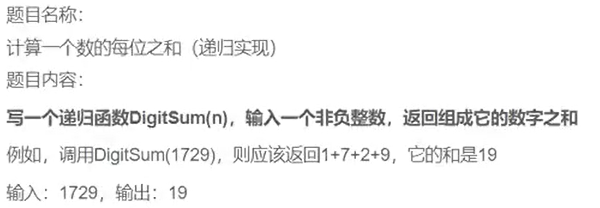循环实现：

``````void DigisSum(unsigned int num)
{
int sum = 0;
while (a > 0)
{
sum += num % 10;
num = num / 10;
}
printf("%d", sum);
}

int main()
{
int num = 0;
scanf("%d", &num);
DigisSum(num);
return 0;
}``````

``````//1729
//DigisSum(172)+1729%10
//DigisSum(17)+(1729/10)%10+1729%10
//DigisSum(1)+((1729/10)/10)%10+(1729/10)%10+1729%10
//num<10时直接相加。即1+7+2+9
int DigitSum(unsigned int num)
{
if (num >= 10)
{
return DigitSum(num / 10) + num % 10;
}
else
{
return num;
}
}

int main()
{
int num = 0;
scanf("%d", &num);
int ret = DigitSum(num);
printf("%d",ret);
return 0;
}``````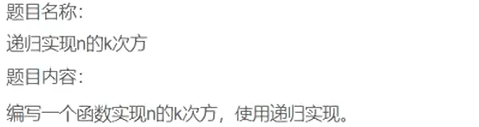``````double Pow(int n, int k)
{
//n^k = n*n^(k-1)
if (k > 0)
{
return n * Pow(n, k - 1);
}
else if (k == 0)
{
return 1;
}
else
{
return (1.0 / Pow(n, -k));  //一个数的负次方即为这个数的正次方的倒数
}
}

int main()
{
int n = 0;
int k = 0;
scanf("%d %d",&n,&k);
double ret = Pow(n, k);
printf("ret = %lf\n", ret);
return 0;
}``````

::: tip n^k = n*n^(k-1) :::Suzhou
Lv1

17

9

0